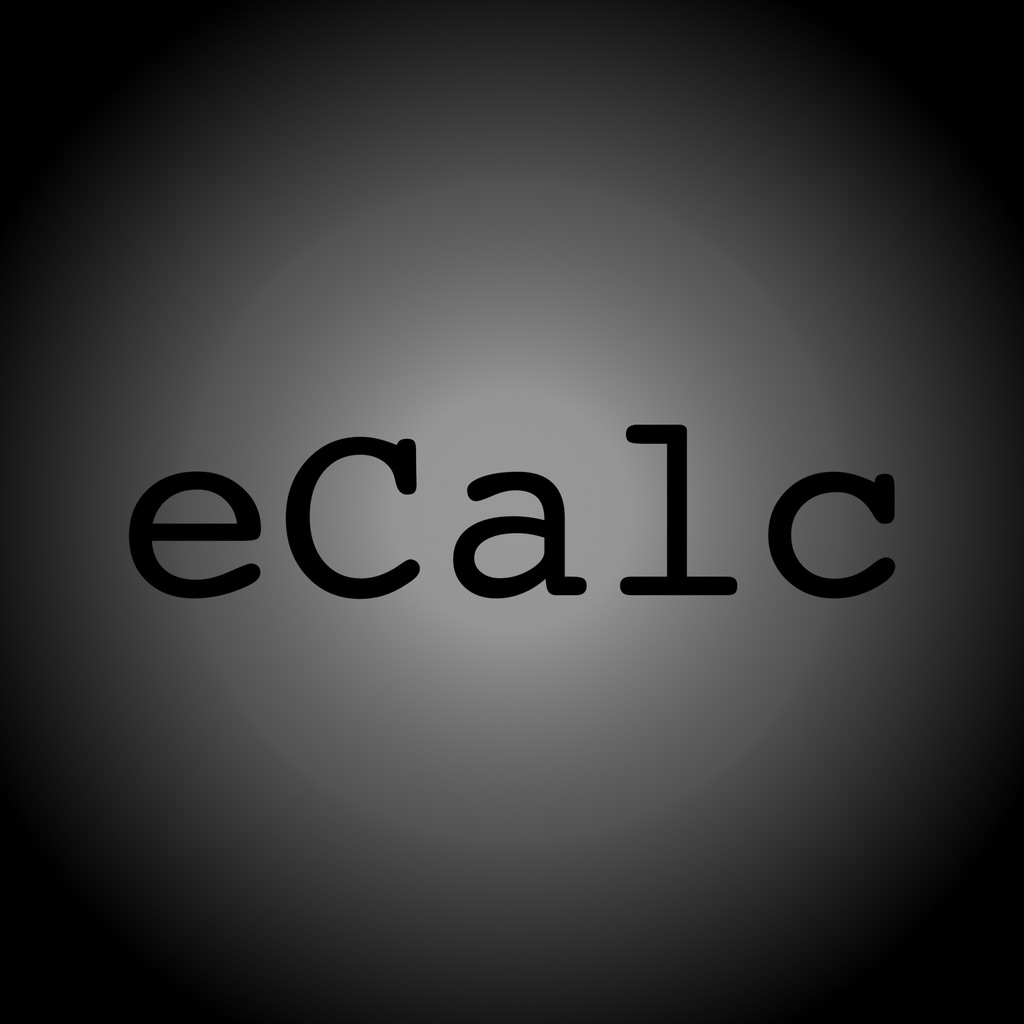EngineerCalc# EngineerCalc

\$1.99
0

0 Ratings

Update Date

2019-03-03

Size

15.8 MB

EngineerCalc Description
EngineerCalc is an app for electrical engineers and hobbyists. At the moment, it includes 4 calculators:

• CapacitorCalc - Calculate the ripple voltage refusing capacitor capacitance and min. voltage for your AC-DC circuit
• InductorCalc - Calculate the inductance, needed wire-length (and resistance of the wire) and Q-value for your diy coil/inductor
• LC-TankCircuit - Calculate the 3rd value of your tank-circuit (capacitance, inductance or frequency)
• Ohm'sLawCalc - Calculate the 3rd and/or 4th value of an Ohm's law circuit (voltage, current, resistance or power )

The app supports multiple units which you can change by tapping the unit-label:

For CapacitorCalc:

• Voltage (Volt) - V
• Current (Amper) - A
• Frequency (Herz) - Hz

For InductorCalc:

• Distance (Meter) - µm, mm, cm, m, Km
• Resistance (Ohm) - pΩ, nΩ, µΩ, mΩ, Ω, KΩ, MΩ, GΩ
• Frequency (Herz) - Hz, KHz, MHz, GHz
• Inductance (Henry) - pH, nH, µH, mH, H, KH, MH, GH

For LC-TankCircuit:

• Capacitance (Farad) - pF, nF, µF, mF, F, KF, MF, GF
• Inductance (Henry) - pH, nH, µH, mH, H, KH, MH, GH
• Frequency (Herz) - Hz, KHz, MHz, GHz

For Ohm'sLawCalc:

• Voltage unit (Volt) - pV, nV, µV, mV, V, KV, MV, GV
• Current unit (Amper) - pA, nA, µA, mA, A, KA, MA, GA
• Resistance unit (Ohm) - pΩ, nΩ, µΩ, mΩ, Ω, KΩ, MΩ, GΩ
• Power unit (Watt) - pW, nW, µW, mW, W, KW, MW, GW

If you have an development suggestion, want to request an unit support or want to report a bug, you can get in touch with me trough my website https://ssl-saario.xyz/AppFiles/html/eCalcHelp.html
EngineerCalc 1.0.3 Update
2019-03-03 Version History
More